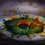# Suggestions for Sharers

The reason why I'm writing this note, is that there are some things which people (especially the people that write problems and solutions) should know. This can really reduce the need of edits by Moderators.

Together, we can make it clearer and hence, more enjoyable.

$\color{#D61F06}{\mathbf{1.}}$ Please try to use $\LaTeX$ mathematical formatting. You can find the guidelines while posting the problem, as shown in the picture that follows, and also hereimg

$\color{#D61F06}{\mathbf{2.}}$ Do $\textbf{NOT}$ wrap all of your question in the \ ( ....... \ ) . Wrap ONLY the Math terms in the \ ( ...... \ )

I've seen people who post something like

which appears as

$Find \quad the \quad value \quad of \quad the \quad integral \quad \int_0^1 x^7 dx$

$\bullet$ They use the '\quad' for separation between the words,because the \ ( ........ \ ) wrapping doesn't take into account the spaces .

$\bullet$ The more preferable way for this is, write only the math term in \ ( ..... \ ) and it will appear as

Find the value of the integral $\int_0^1 x^7 dx$

$bullet$ This way, the paragraph and alignment formatting will be automatically considered, for example

$My\quad name\quad is\quad Aditya\quad and\quad I\quad love\quad solving\quad problems\quad on\quad Brilliant.org\quad very\quad much.\quad See\quad the \quad math\quad \dfrac{\sqrt{1234}}{\sqrt{4321}}$

In the above display, you are having to scroll to read it all (If not here, in problems you surely have to scroll).

$\bullet$ But if the wrapping is only around the math terms, then It will appear as

My name is Aditya and I love solving problems on Brilliant.org very much. See the math $\dfrac{\sqrt{1234}}{\sqrt{4321}}$

$\color{#D61F06}{\mathbf{3.}}$ Please use the symbol $\dbinom{n}{r}$ instead of $^nC_r$.

$\bullet$ The $\LaTeX$ code is \binom{n}{r}, and for clearer display , use \dbinom{n}{r}

$\bullet$ Use this, because when the binomial coefficient is preceded by some number, one could misread $6^nC_r$ , which is actually $6\times ^nC_r$, as $6^n\times C_r$ .

$\color{#D61F06}{\mathbf{4.}}$ Whenever it's difficult or lengthy to explain the question by words (mostly in Geometry Problems) , give a diagram with the question. You may use softwares like Geogebra or Asymptote

$\color{#D61F06}{\mathbf{5.}}$ Whenever an image is taken from some source (like some website or somewhere), at times it may be subject to copyright, so just give the source of the image at bottom of the question (preferably a link to the source).

$\bullet$ Write image credit - < source > preceded by 6 '#' signs. For example, writing $\text{\#\#\#\#\#\# Image Credit wikipedia}$ will appear as

###### Image Credit Wikipedia

$\bullet$ This way, write the Credit at the bottom of your question. An example can be seen in this problem

Further, the more issues i notice will be added here, and additions are welcome!6 years, 9 months ago

This discussion board is a place to discuss our Daily Challenges and the math and science related to those challenges. Explanations are more than just a solution — they should explain the steps and thinking strategies that you used to obtain the solution. Comments should further the discussion of math and science.

When posting on Brilliant:

• Use the emojis to react to an explanation, whether you're congratulating a job well done , or just really confused .
• Ask specific questions about the challenge or the steps in somebody's explanation. Well-posed questions can add a lot to the discussion, but posting "I don't understand!" doesn't help anyone.
• Try to contribute something new to the discussion, whether it is an extension, generalization or other idea related to the challenge.

MarkdownAppears as
*italics* or _italics_ italics
**bold** or __bold__ bold
- bulleted- list
• bulleted
• list
1. numbered2. list
1. numbered
2. list
Note: you must add a full line of space before and after lists for them to show up correctly
paragraph 1paragraph 2

paragraph 1

paragraph 2

[example link](https://brilliant.org)example link
> This is a quote
This is a quote
    # I indented these lines
# 4 spaces, and now they show
# up as a code block.

print "hello world"
# I indented these lines
# 4 spaces, and now they show
# up as a code block.

print "hello world"
MathAppears as
Remember to wrap math in $$ ... $$ or $ ... $ to ensure proper formatting.
2 \times 3 $2 \times 3$
2^{34} $2^{34}$
a_{i-1} $a_{i-1}$
\frac{2}{3} $\frac{2}{3}$
\sqrt{2} $\sqrt{2}$
\sum_{i=1}^3 $\sum_{i=1}^3$
\sin \theta $\sin \theta$
\boxed{123} $\boxed{123}$

Sort by:

- 6 years, 9 months ago

- 6 years, 9 months ago

Huh... You need to upload them somewhere first, like imgur.com

Then you need to do this.

 1  ![image dexcription](http://example.com/kabooblydoo.jpg) 

- 6 years, 9 months ago

And be sure to use the direct link to the image and that it ends in a pic extension like jpg.

- 6 years, 9 months ago

Can you please share how to use colored fonts in questions? It might be helpful in some way! @Aditya Raut

- 6 years, 8 months ago

I've written a full note on that! Bingo! Attractive Solutions

- 6 years, 8 months ago

Nice introduction to beginners!

- 6 years, 4 months ago

- 6 years, 9 months ago

Indeed. Together, we can help to enhance the overall experience on Brilliant.

- 6 years, 9 months ago